# Team:Oxford/Model/Further-Development

## Part I: Spatial Stochastic Model

### Assumptions

#### To obtain qualitative results from the spatial simulations, we made the following assumptions:

• As the size of bacterial cells is minute relative to the intestine, we can ignore the curvature of the intestinal wall upon which the bacterial cells thrive.
• Kinetic rate constants are estimated such that 90% of the C. difficile population would be upregulated by 2-3 AIP molecules per cell.
• The efficiency of the AgrAC two-component regulatory system in our L. reuteri would be equal to that in C. difficile

#### We wanted to model the following three scenarios:

1. C. difficile and L. reuteri colonies are uniformly mixed.
2. C. difficile and L. reuteri colonies are separated but placed adjacently.
3. C. difficile and L. reuteri colonies are spatially separated.

### For a single signalling system:

Kinetic Rate Equation Kinetic Rate Constant Kinetic Rate Constant Value Modelled
$$C. difficile_{downregulated} \to C. difficile_{downregulated} + AIP$$ $$k_S$$ $$10^{3} \ M \ S^{-1}$$
$$AIP \to [NULL]$$ $$\delta_AIP$$ $$10^{2} \ S^{-1}$$
$$C. difficile_{downregulated} + AIP \to C. difficile_{upregulated}$$ $$\phi_c$$ $$10^{6} \ M^{-1} \ S{-1}$$
$$L. reuteri_{downregulated} + AIP \to L. reuteri_{upregulated}$$ $$\phi_l$$ $$10^{6} \ M^{-1} \ S{-1}$$

### Diffusion Constants

Species Diffusion Rate Constant Value Modelled
$$AIP$$ $$10^{-9} \ cm^{2} \ s^{-1}$$

### C. difficile and L. reuteri colonies assumed uniformly mixed.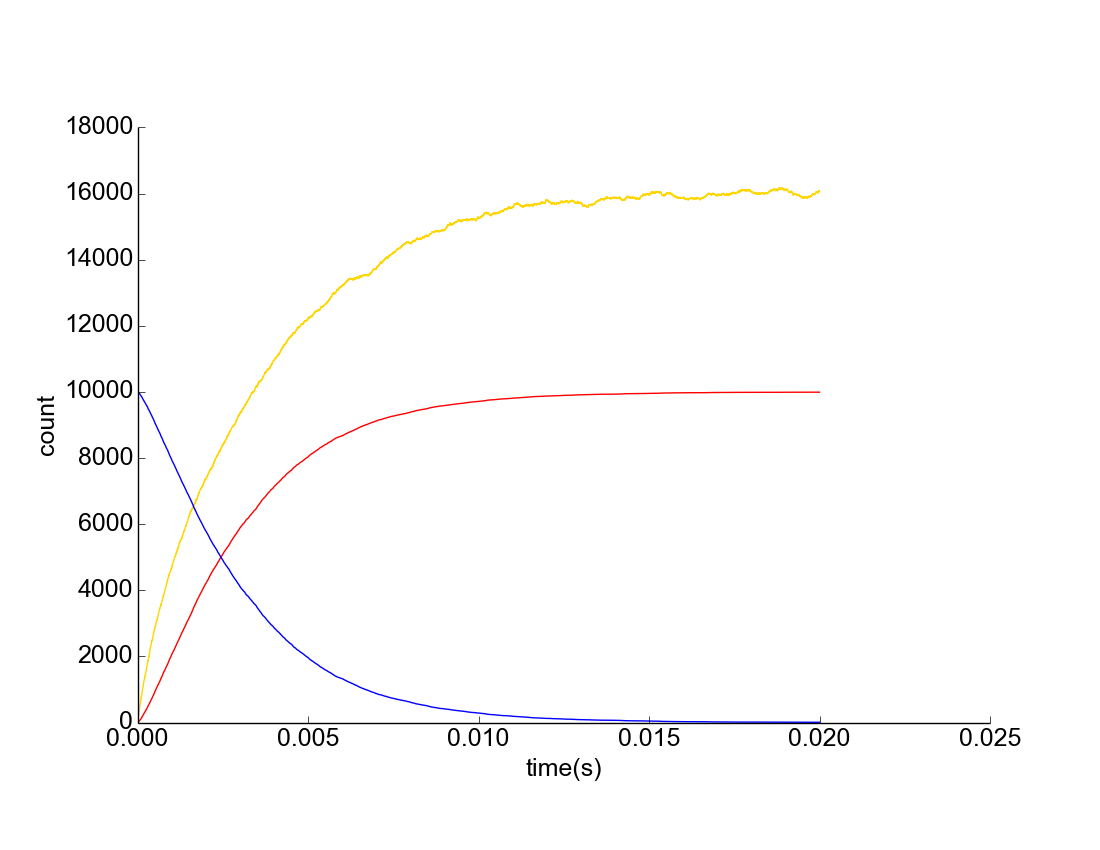Figure 1. Upregulation of C. difficile Assuming Uniform Mixture. Yellow represents AIP concentration, Blue represents downregulated C. difficile population, Red represents upregulated C. difficile population.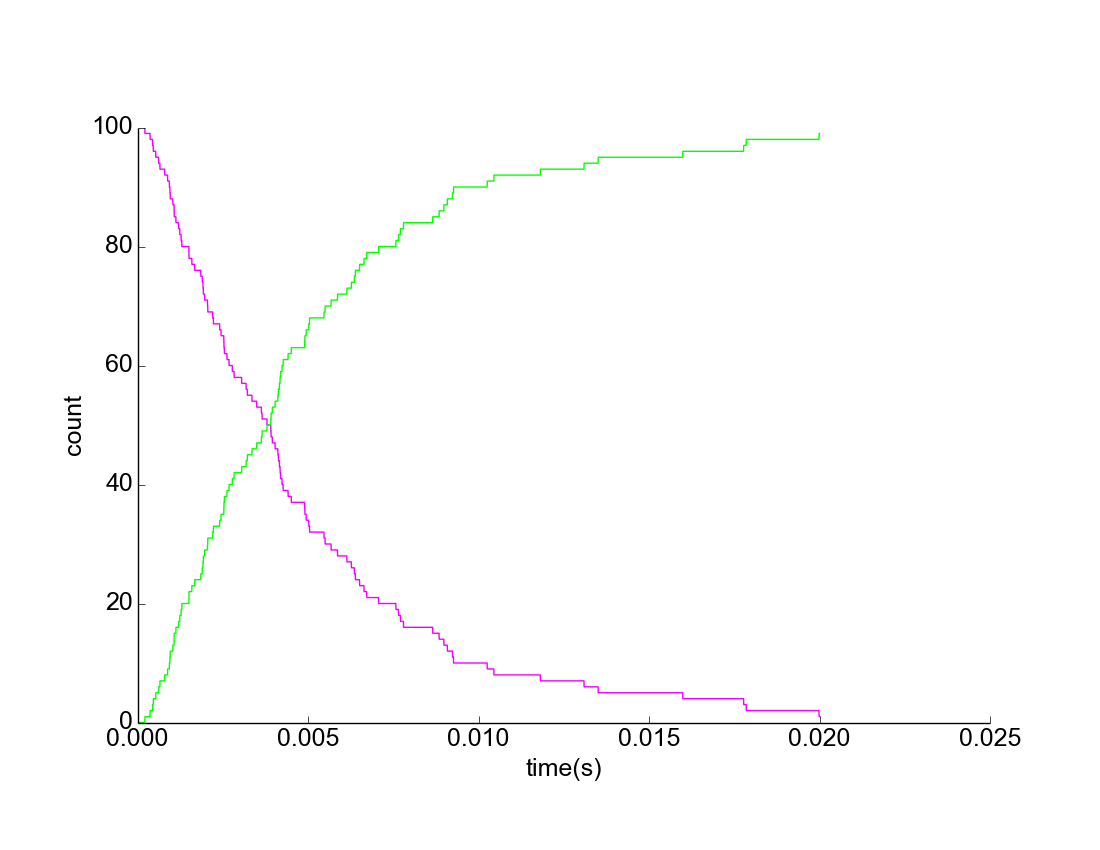Figure 2. Upregulation of L. reuteri Assuming Uniform Mixture. Purple represents downregulated L. reuteri population; Green represents upregulated L. reuteri population.

### C. difficile and L. reuteri colonies assumed adjacent to each other.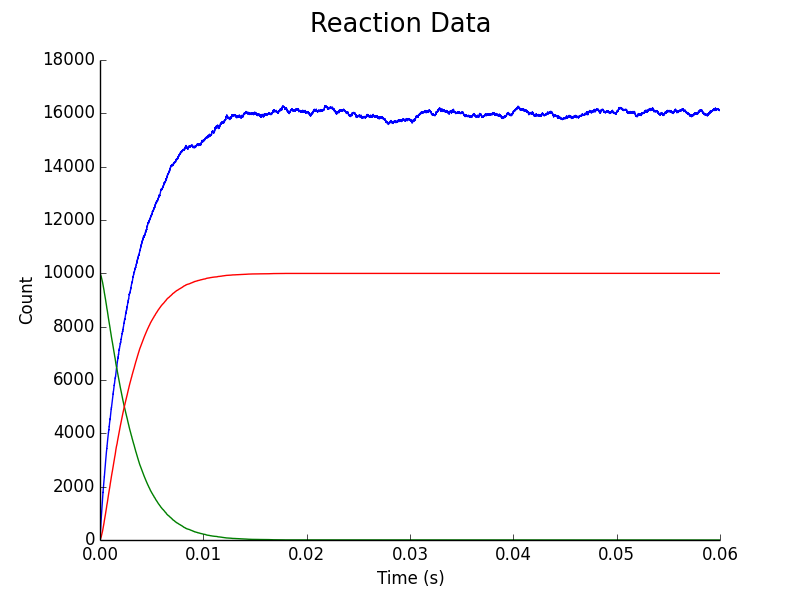Figure 3. Upregulation of C. difficile assuming C. difficile & L. reuteri Colonies Adjacent. Blue represents AIP concentration, Green represents downregulated C. difficile population, Red represents upregulated C. difficile population.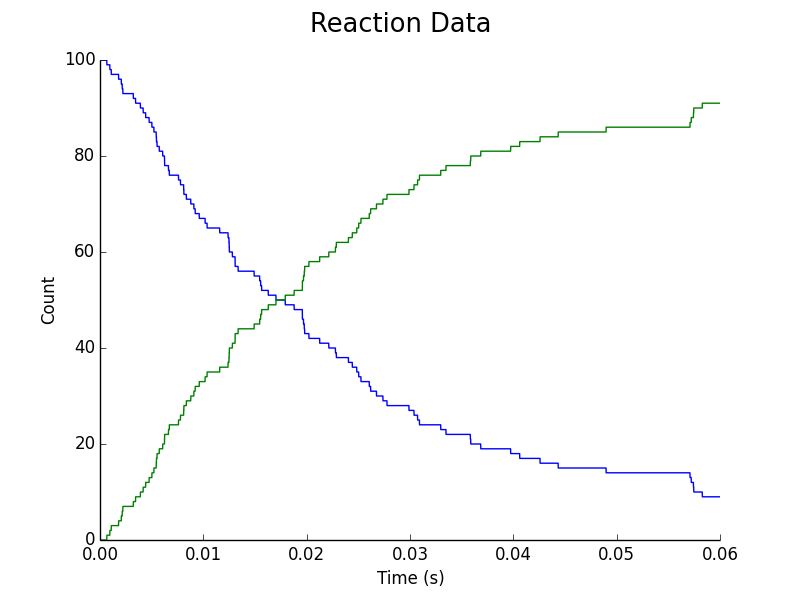Figure 4. Upregulation of L. reuteri assuming C. difficile & L. reuteri Colonies Adjacent. Blue represents downregulated L. reuteri population; Green represents upregulated L. reuteri population.

### C. difficile and L. reuteri colonies assumed spatially separated.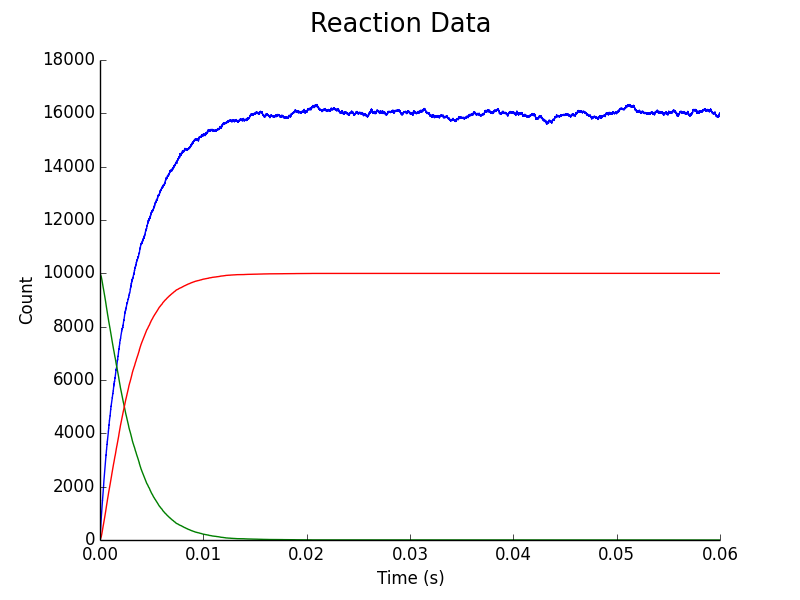Figure 5. Upregulation of C. difficile assuming C. difficile & L. reuteri colonies are separated by a fixed distance in space. Blue represents AIP concentration; Green represents downregulated C. difficile population; Red represents upregulated C. difficile population.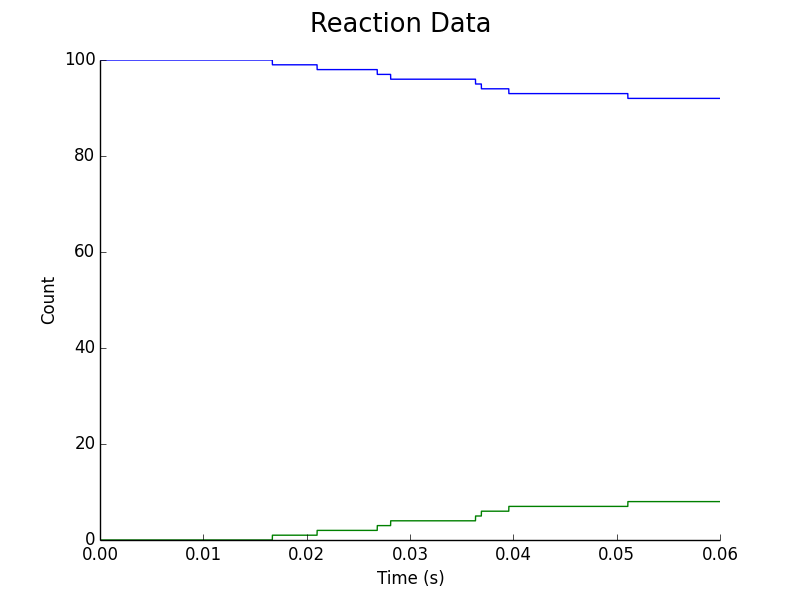Figure 6. Upregulation of L. reuteri assuming C. difficile & L. reuteri colonies are separated by a fixed distance in space. Blue represents downregulated L. reuteri population, Green represents upregulated L. reuteri population.

## Part II: Secondary Amplification System Spatial Model

### Let the secondary amplification signalling molecule be denoted by S. For the secondary amplification system:

Kinetic Rate Equation Kinetic Rate Constant Kinetic Rate Constant Value Modelled
$$L. reuteri_{downregulated} \to L. reuteri_{downregulated} + S$$ $$k_S$$ $$10^{3} \ M \ s^{-1}$$
$$S \to [NULL]$$ $$\delta_S$$ $$10^{2} \ s^{-1}$$
$$L. reuteri_{downregulated} + S \to L. reuteri_{upregulated}$$ $$\gamma$$ $$10^{6} \ M^{-1} \ s^{-1}$$

### Diffusion Constants

Species Diffusion Rate Constant Value Modelled
$$AIP$$ $$10^{-9} \ cm^{2} \ s^{-1}$$

### Results

#### On rerunning the simulation when the C. difficile and L. reuteri colonies are separated by a fixed distance in space, the following graph was obtained: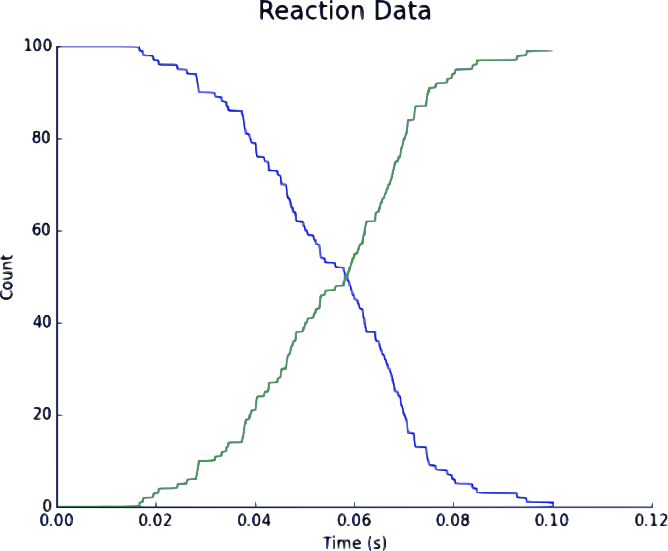Figure 1. Upregulation of L. reuteriwith a Secondary Signalling System when C. difficile & L. reuteri are separated by a fixed distance in space. Blue represents downregulated L. reuteri population; Green represents upregulated L. reuteri population.

## Part III: Secondary Signalling System Deterministic Model

### Assumption

1. Signal X and receptor Y expression causes negligible cellular stress
2. Kinetic rates of the secondary amplification system are identical to those for the AgrAC two-component signalling system as in the Model Designpage.
3. Signal X and receptor Y can freely diffuse through cell membranes.
4. The upregulation profile due to signal-receptor complex XY can be approximated using an upregulation cut-off concentration as previously assumed for the AgrAC two-component signalling system.
5. A single molecule of signal-receptor complex XY is sufficient to cause upregulation. Consequently, the decrease in XY concentration can be ignored.

#### Species Key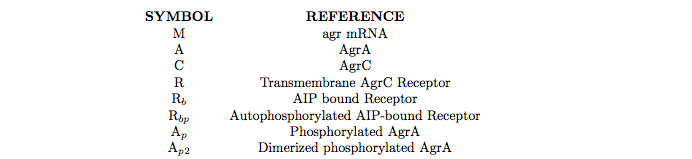### Transcription of Signalling Molecule X & Receptor Y

#### As mRNA transcription is triggered by dimerised, phosphorylated AgrA, the relevant kinetic equations are written as:

Kinetic Rate Equation Kinetic Rate Constant Kinetic Rate Constant Value Modelled
$$NULL \to M_{xy}$$ $$m_2 \ (if [Ap_2] \geq 6.514 \times 10^{-9} \ M); \\ 0 \ (if [Ap_2] \lt 6.514 \times 10^{-9} \ M)$$ $$8.33 \ M \ hour^{-1} \ cell^-1$$

### Translation of Signalling Molecule & Receptor Y

Kinetic Rate Equation Kinetic Rate Constant Kinetic Rate Constant Value Modelled
$$M_{xy} \to X$$ $$v$$ $$1.67 \times 10^{-7} \ minutes^{-1}$$
$$M_{xy} \to Y$$ $$v$$ $$1.67 \times 10^{-7} \ minutes^{-1}$$

### Ligand Binding of X to Receptor Y & Subsequent Formation of Transcription Factor

Kinetic Rate Equation Kinetic Rate Constant Kinetic Rate Constant Value Modelled
$$X + Y \to XY$$ $$k_{ir}$$ $$0.0167 \ M^{-1} \ minutes^{-1}$$

### Upregulation of Cell by Transcription Factor of Secondary Signalling System

Kinetic Rate Constant Kinetic Rate Constant Value Modelled
$$P$$ $$1 \ (if XY \geq 0.25 \times 10^{-5} \ M) \ or \ 0 \ (if XY \lt 0.25 \times 10^{-5} \ M)$$

#### Such a upregulation cutoff of XY was deliberately chosen so that small concentrations of XY transcription factor can upregulate the cell.

Kinetic Rate Equation Kinetic Rate Constant Kinetic Rate Constant Value Modelled
$$X \to NULL \ (where X=M_{xy}, X, Y)$$ $$\delta_X$$ $$0.0556 \ hour^{-1}$$

#### Differential Equations

Concentration Modelled Differential Equation Process Modelled
mRNA for Signaling Molecule X & Receptor Y $$\frac {dM_{xy}}{dt} = m_2 - \delta_{M_{xy}} \times M_{xy}$$ Transcription + Degradation
Signalling Molecule X $$\frac {dX}{dt} = v \times M_{xy} - k_{ir} \times X \times Y - \delta_X \times X$$ Translation + Ligand Binding + Degradation
Signalling Molecule Y $$\frac {dY}{dt} = v \times M_{xy} - k_{ir} \times X \times Y - \delta_Y \times Y$$ Translation + Ligand Binding + Degradation
Ligand-Receptor Complex XY $$\frac {dXY}{dt} = k_{ir} \times X \times Y - \delta_{XY} \times XY$$ Ligand Binding + Degradation

### Results

#### To see how the transcription factor changes during upregulation and downregulation, we input an AIP concentration of 1 nM at 350 hours, and removed it at 650 hours.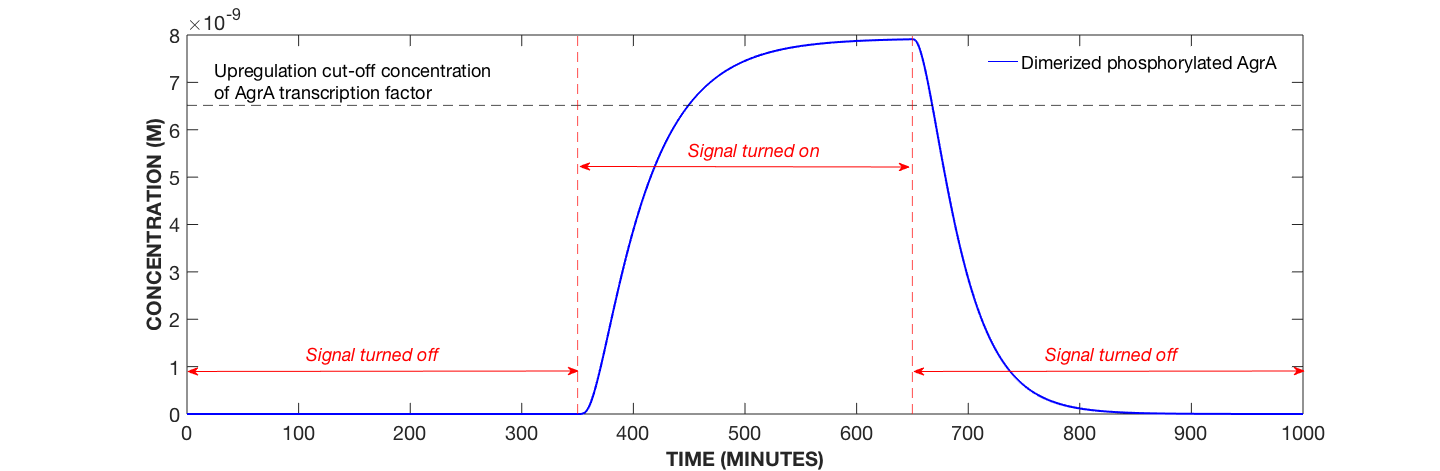Figure 1. Dynamics of Dimerised, Phosphorylated AgrA Protein with Secondary Amplification System, AIP Concentration Modulated. Specifically, AIP added at 350 minutes and removed at 650 minutes.

#### The concentration of dimerized phosphorylated AgrA starts dropping immediately after the signal is taken away. It takes less than an hour to drop below the upregulation cut-off concentration.

\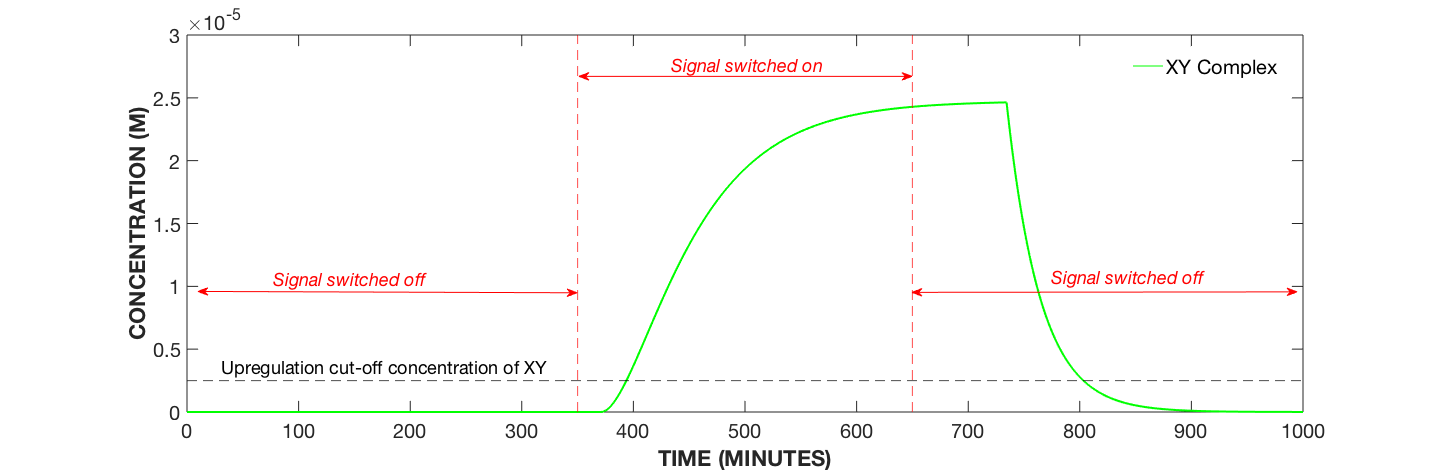Figure 2. Dynamics of Signal-Receptor Complex XY with Secondary Amplification System, AIP Concentration Modulated. Specifically, AIP added at 350 minutes and removed at 650 minutes.

#### The concentration of the XY complex increases even after the signal has been switched off. This is because XY complex production is dependant on the upregulation of the cell, which in turn is controlled by the concentration by dimerized phosphorylated AgrA. As AgrA concentration takes some time to drop below it’s upregulation cut-off concentration, the XY complex is produced even after the signal is switched off.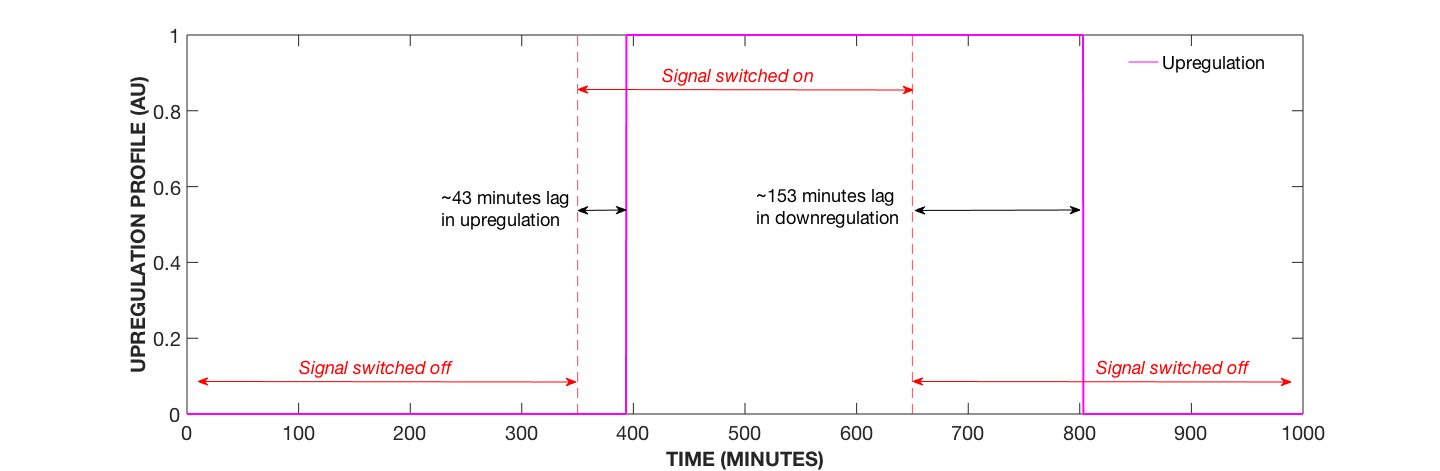Figure 3. Upregulation Profile with Secondary Amplification System, AIP Concentration Modulated. Specifically, AIP added at 350 minutes and removed at 650 minutes.

#### The cell remains upregulated for a significant amount of time after the AIP signal switched off. The time lag is caused by:

1. Time taken by dimerized phosphorylated concentration to drop below its upregulation cut-off concentration.
2. Time taken by XY complex concentration to drop below its upregulation cut-off concentration.

## References

Reference
Stiles, JR, et al. (1996). Miniature endplate current rise times < 100 μs from improved dual recordings can be modeled with passive acetylcholine diffusion from a synaptic vesicle. Proc. Natl. Acad. Sci. USA 93:5747-5752.
Jabbari, Sara, et al. “Mathematical Modelling of the Agr Operon in Staphylococcus Aureus.” Journal of Mathematical Biology, vol. 61, no. 1, 2009, pp. 17–54., doi:10.1007/s00285-009-0291-6.
Stiles, JR, and Bartol, TM. (2001). Monte Carlo methods for simulating realistic synaptic microphysiology using MCell. In: Computational Neuroscience: Realistic Modeling for Experimentalists, ed. De Schutter, E. CRC Press, Boca Raton, pp. 87-127.
Kerr R, Bartol TM, Kaminsky B, Dittrich M, Chang JCJ, Baden S, Sejnowski TJ, Stiles JR. (2009). Fast Monte Carlo Simulation Methods for Biological Reaction-Diffusion Systems in Solution and on Surfaces. SIAM J. Sci. Comput., 30(6):3126-3149.